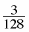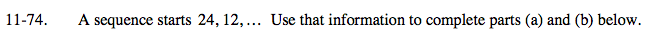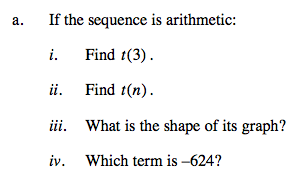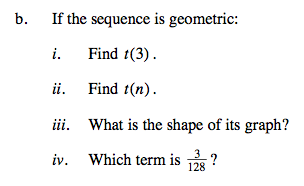### Home > A2C > Chapter Ch11 > Lesson 11.2.3 > Problem11-74

11-74.
1. A sequence starts 24, 12, … Use that information to complete parts (a) and (b) below. Homework Help ✎

1. If the sequence is arithmetic:

1. Find t(3).

2. Find t(n).

3. What is the shape of its graph?

4. Which term is −624?

2. If the sequence is geometric:

1. Find t(3).

2. Find t(n).

3. What is the shape of its graph?

4. Which term isAdd (or subtract) the same amount again.

t(n) = 36 − 12n

Try graphing the sequence as a function.

Substitute −624 for t(n) and solve for n.Multiply (or divide) by the same amount again.

$t(n)=48\left(\frac{1}{2}\right)^{n}$

Try graphing the sequence as a function.

$\text{Substitute }\frac{3}{128}\text{ for } t(n)\, \text{and solve for n.}$